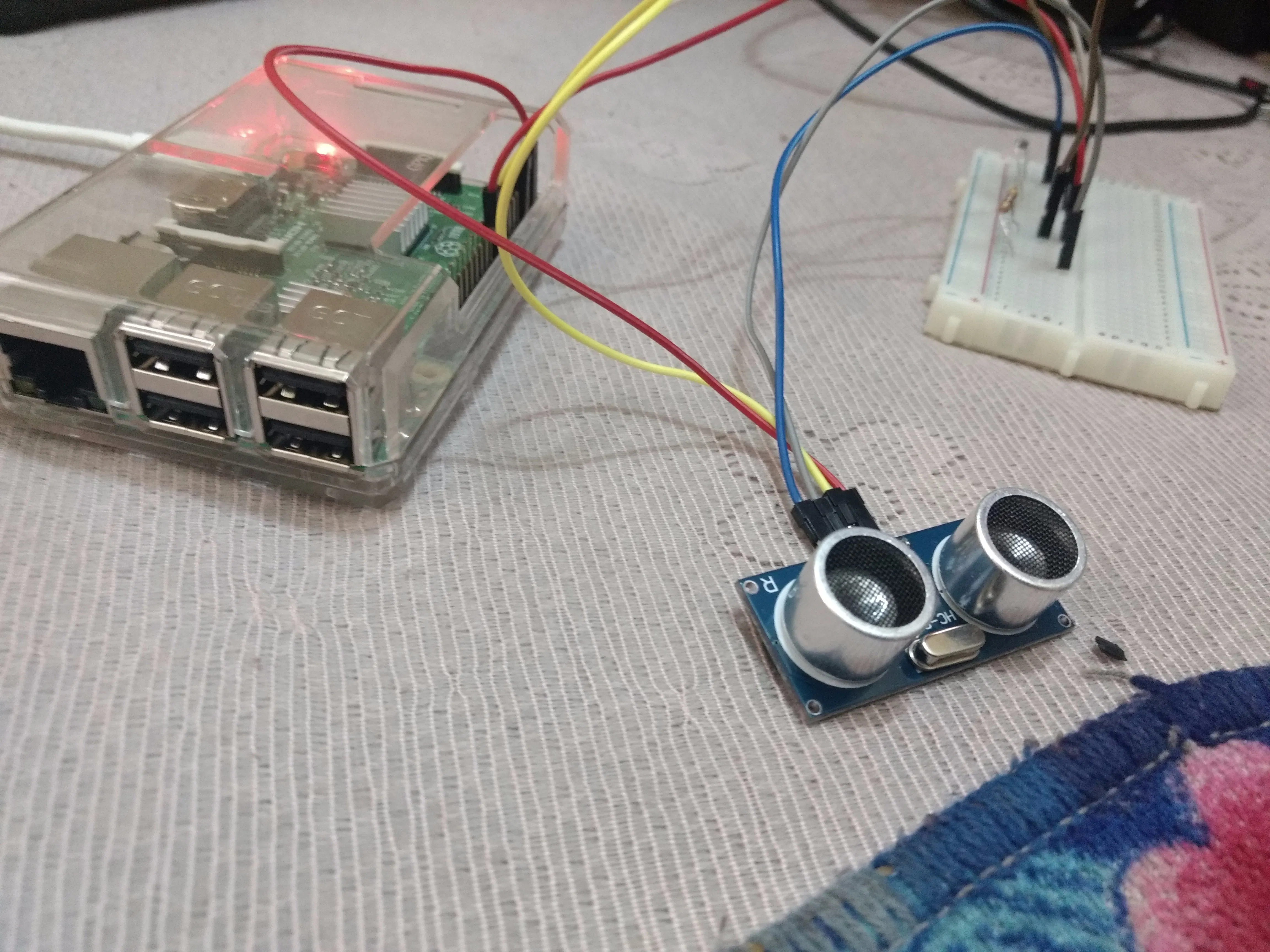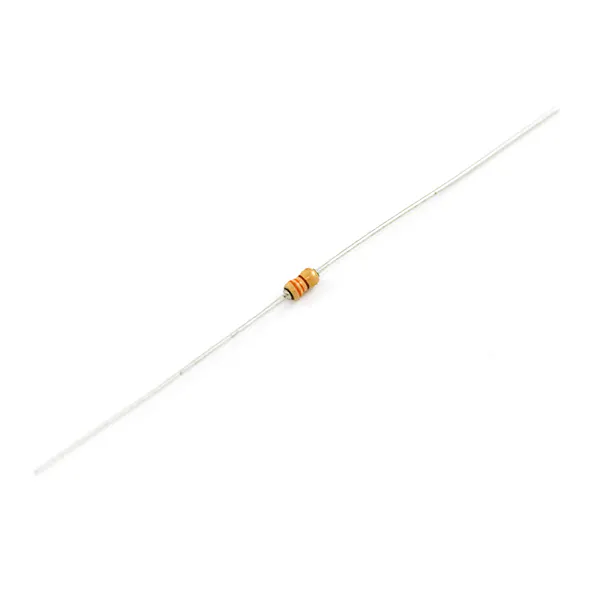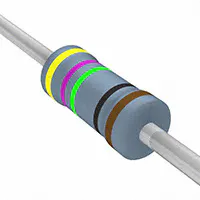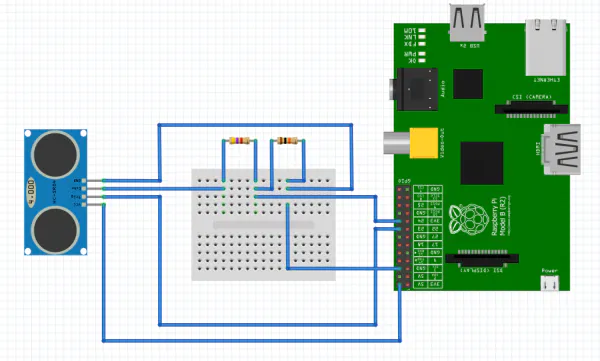Published

Distance Calculation with Ultrasonic Sensor

Ultrasonic distance sensors are designed to measure distance between the source and target using ultrasonic waves.

BeginnerFull instructions provided1 hour23,407Things used in this project

Hardware componentsRaspberry Pi 3 Model B
×1Resistor 330 ohm
×1Resistor 475 ohm
×1×1Jumper wires (generic)
×1Ultrasonic Sensor - HC-SR04 (Generic)
×1

Schematics

Connection DiagramCode

dist-sensor.py

Python
import RPi.GPIO as GPIO                    #Import GPIO library
import time                                #Import time library
GPIO.setmode(GPIO.BCM)                     #Set GPIO pin numbering

TRIG = 23                                  #Associate pin 23 to TRIG
ECHO = 24                                  #Associate pin 24 to ECHO

print "Distance measurement in progress"

GPIO.setup(TRIG,GPIO.OUT)                  #Set pin as GPIO out
GPIO.setup(ECHO,GPIO.IN)                   #Set pin as GPIO in

while True:

GPIO.output(TRIG, False)                 #Set TRIG as LOW
print "Waitng For Sensor To Settle"
time.sleep(2)                            #Delay of 2 seconds

GPIO.output(TRIG, True)                  #Set TRIG as HIGH
time.sleep(0.00001)                      #Delay of 0.00001 seconds
GPIO.output(TRIG, False)                 #Set TRIG as LOW

while GPIO.input(ECHO)==0:               #Check whether the ECHO is LOW
pulse_start = time.time()              #Saves the last known time of LOW pulse

while GPIO.input(ECHO)==1:               #Check whether the ECHO is HIGH
pulse_end = time.time()                #Saves the last known time of HIGH pulse

pulse_duration = pulse_end - pulse_start #Get pulse duration to a variable

distance = pulse_duration * 17150        #Multiply pulse duration by 17150 to get distance
distance = round(distance, 2)            #Round to two decimal points

if distance > 2 and distance < 400:      #Check whether the distance is within range
print "Distance:",distance - 0.5,"cm"  #Print distance with 0.5 cm calibration
else:
print "Out Of Range"                   #display out of range

Credits

Arbaz Hussain

0 projects • 11 followers
cat /etc/arbaz | grep Machine Learning && Bug Hunter